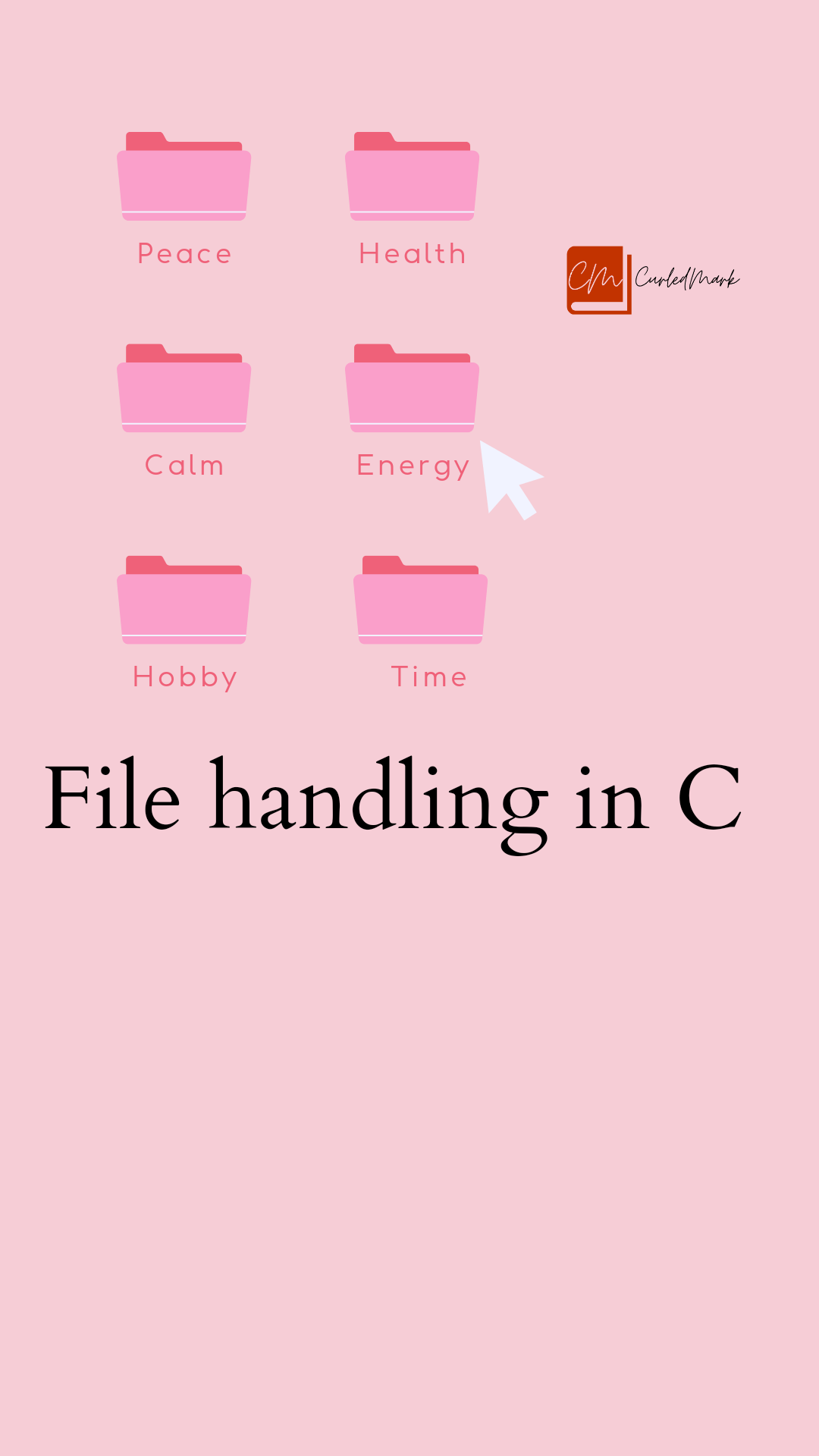January 31, 2023# The largest number Program in an array in C programming

## Explanation of the largest number program logic

In the below pictorial representation, there is an array of 8 elements. If a program states that we need to find the greatest number from these 8 numbers, how do we do that? In a layman’s way, we can simply compare the first number in an array with the rest of the numbers.

First, we will consider 100 which is the first element of the array, and compare it with the second which is 50. Here we can see that 100 is greater than 50. So, we can move to the next number. And will compare 100 with 5. 100 is also greater than 5. Moving to the next number, that is 130. Now we can see that 100 is less than 130. Now in the RAM 130 is stored and it will be compared with the rest of the elements.

It is greater than 40. Then it moves to the next number. 130 is greater than 79. Again when we compare it with 1500, it is greater than 130. Now, 1500 is the greatest. Still, there is another number to compare with. It compares with 10 and stays the greatest number. This is how the comparison is done.

If we write this in a program, it should be in a simple way to decode at once right? So, let’s write an array program to find the greatest number of all the numbers in an array.## Logic

int large = x;

y=1;

While(y<=7)

{

if(x[y]>large)

{

large=x[y];

}

y++;

}

## Write a Program to accept 8 numbers. Find and print the largest number.

`#include<stdio.h>`

`int main()`

`{`

`int x,int y=0,large;`

`While(y<=7)`

`{ `

`Printf("Enter a number:");`

`Scanf("%d",&x[y]);`

` y++:`

`} `

`y=1,large=x;`

`While(y<=7)`

`{`

`if(x[y]>large)`

`{`

`large=x[y];`

`}`

`y++;`

`}`

`Printf("The number is%d\n",large); `

`return 0;`

`} `

## Explanation of the above code

Firstly we have declared the array size as 8 as x. Then the number which will be the greatest is large. Under the first section of the while, we have given the input from the keyboard. 8 numbers we will type in the output screen to get the large number output.

This is for the first section of the while loop. Before the second while loop, we have declared the number array size y as 1 and large equals x which will store the first number from the array in the large. That is large =x where x is 100(You can refer to the above pictorial representation).

Likewise, it will be x,x,x, as y increments. As the logic says it will compare one by one in the “if statement” written in the program and stored in the large variable. Finally, it prints the large number from the array and we get the output.

## Output

`Enter a number: 100`

`Enter a number: 50`

`Enter a number: 5`

`Enter a number: 130`
``` Enter a number: 40```

`Enter a number: 79`
``` Enter a number: 1500```
``` Enter a number: 10```

The number is 1500

## Conclusion

So, here is all about the greatest number in the array in C. We hope you find this implementation alternative. Stay tuned for the upcoming blogs.

The Da Vinci Code by Dan Brown Summary

The Book Of Five Rings Summary

The Blue Umbrella

How to Talk to Anyone by Leil Lowndes

Jonathan Livingston Seagull Book Summary

Your Brain on PORN Book Summary by Gary Wilson

THE IMMORTALS OF MELUHA By Amish Tripathi full summary

Eleven Minutes by Paulo Coelho Summary

Hyperfocus by Chris Bailey Summary

Black Beauty by Anna Sewell Summary

You Can Heal Your Life by Louise Hay Summary

ATTITUDE IS EVERYTHING BY JEFF KELLER SUMMARY

THE BIOGRAPHY OF SWAMI VIVEKANANDA

THE SHAWSHANK REDEMPTION EXPLAINED

Turtles All the Way Down by John Green Story Explained

Essentialism The Disciplined Pursuit of Less by Greg McKeown Summary

The One from the Stars by Keshav Aneel

The Miracle Morning Guaranteed to Transform Your Life (Before 8AM)

The Little Prince Book Summary by Antoine de Saint-Exupery

The Courage to Be Disliked Book Summary

Prime, reverse,power Programs in C Programming

IF ELSE Block in C Programming || IF ELSE BLOCK

Armstrong number and arrays in C Programming

Looping and printing number series in C

IF ELSE Block in C Programming || IF ELSE BLOCK

Operators and accepting input from Keyboard in C Programming

Fundamentals of C Programming in simple way

#### You may have missed#### RIYA SHUKLA, DIVYANSH DWVEDI & CHITRANSH RAAJ TO SHARE SCREEN IN FILM PULLU